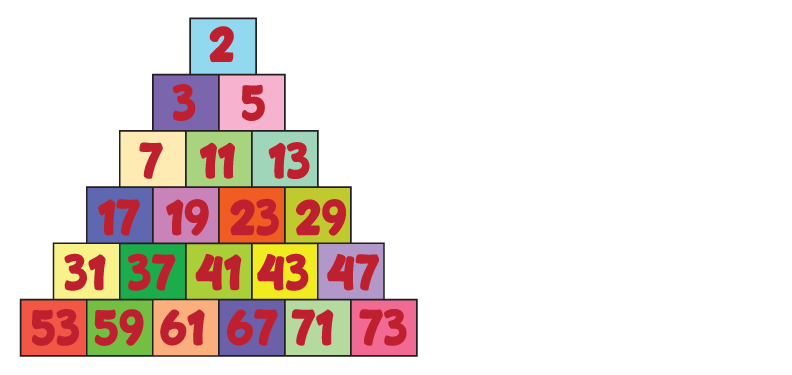What is a prime number?

A prime number is a number greater than 1 that cannot be made by multiplying other numbers.

Example:

You cannot multiply two numbers to get 3. 3 is a prime number.

4 is not a prime number, it’s a composite number. You can multiply 2 x 2 to get 4.

1 is not a prime number or a composite number.

Let's have a look at the number 13. 13 can only be obtained by multiplying 1 x 13 BUT as the number 1 cannot be counted, 13 is in fact a prime number.

List of prime numbers to 10: 2, 3, 5 and 7.

Here are some more prime numbers: